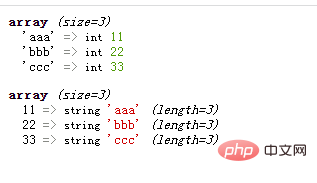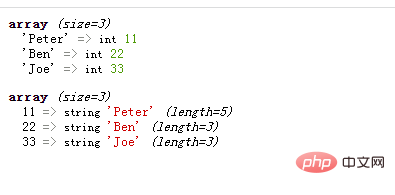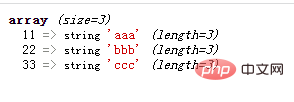# php怎么将数组value转为key

3种方法：1、用“array_flip(\$arr)”语句；2、用“array_combine(\$arr,array_keys(\$arr))”语句；3、用“foreach(\$arr1 as \$k=>\$v){\$arr2[\$v]=\$k;}”语句。php将数组value转为key，也就是将数组中键和值互相转换位置。下面介绍3种方法：

array_flip()函数可以交换数组中的键和值

```<?php
\$arr1=array("aaa"=>11,"bbb"=>22,"ccc"=>33);
var_dump(\$arr1);
\$arr2=array_flip(\$arr1);
var_dump(\$arr2);
?>```• 使用array_keys()获得数组中的键名，返回键名数组

• 使用array_combine()将原数组作为键名，将键名数组作为键值，合并成一个新数组

```<?php
\$arr=array("Peter"=>11,"Ben"=>22,"Joe"=>33);
var_dump(\$arr);
\$keys=array_keys(\$arr);
var_dump(array_combine(\$arr,\$keys));
?>``````<?php
\$arr1=array("aaa"=>11,"bbb"=>22,"ccc"=>33);
\$arr2=array();
foreach(\$arr1 as \$k=>\$v){
\$arr2[\$v]=\$k;
}
var_dump(\$arr2);
?>```1/1